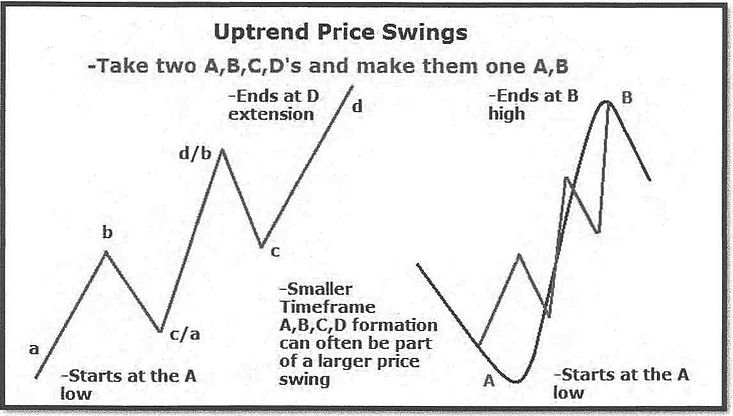top of page

# FIBONACCI

Forex traders uses Fibonacci to pin point where to place for the market entry taking profits at a stop loss orders. Fibonacci levels are used to identify and trade off of support and resistance levels.

Fibonacci Play

Fibonacci is one of the most famous names in mathematics. This would come as a surprise to Leonardo Pisano, the mathematician who is famously know by that name. And he might have been equally surprised that he has been immortalized in the famous sequence - O, 1, 1, 2, 3, 5, 8, 13, (adding the previous two numbers together will give you the next in the sequence) - rather than for what is considered his far greater mathematical achievement - helping to popularize the modern number system in the Latin speaking world.

The Fibonacci number sequence is what constructs sacred geometrical patterns. Everything in the universe is constructed in a spiral fashion. Fibonacci sequences are what construct nature and even the genetic and physical make up of the human body. If you take a pair of next-door Fibonacci numbers and divide the larger by the smaller, you get an approximation to a number called the

golden ratio (1.618). For example: 13 divided by 8 =1.625, 21 divided by 13 = 1.615.

Alongside the geometrical patterns in nature and the relation to this numerical sequence within the human organism, it can also be applied into the markets and utilized in order to find high probability trading setups on a multitude of timeframes.The Fibonacci Levels which you should focus on primarily are:

• Retracement level:  61.8%  Golden Number pullback bounce.

• Retracement level: 78 .6% - Stop-loss level to be placed - 10 PIPs

• Extension levels:  -61.8% + -27% - Target area for trend continuation.Bullish & Bearish Fibonacci

The Fibonacci should be drawn from a significant low point (FIB A) and dragged up towards the next significant high (FIB B). These significant pints are defined by candlestick formations at levels of support/ resistance, once satisfied with the A-B wave the Fibonacci, pull the tool over to the right hand side to allow P.A to play out.When applying Fibonacci, start on the weekly timeframe (in the same direction as the momentum/trend) which should have already been determined throughout the practice of earlier technical phases. Remember that the currency can take a longer period of time to reach the potential D1 + D2 extension levels on the weekly and daily charts and is a primary tool used to determine a clearer directional bias of the overall trend. The H4 & H2 Timeframes are the best for Fibonacci execution...these are the timeframes in which you must incorporate the stop loss rule (15 PIPS above the 78.6 %)Below is a clear example of FIB perfection! This GBP/AUD weekly chart completed a bullish A,B,C,D pattern. The 38.2% FIB retracement level held extremely well, launching the price into new high territory with an almost perfect reaction from the FIB D2 extension level. Once a Fibonacci A, B,C, D has been completed we can now go ahead and draw a new FIB from the FIB C point up to the FIB D point (reversal level). This is ideal for a new FIB wave if the market decides to ~pullback before going even higher.As you can see, once a Fibonacci setup has been completed, a new FIB can be applied from the previous FIB C (Now new FIB A) up to the previous FIB D (Now new FIB B) These points are displayed below (highlighted green) with the new FIB tool displaying the potential FIB retracement levels for a potential new higher low to be formed triggering a bullish trend continuation (With targets projected to the new FIB d extension).The below example is a bullish A,B,C,D H4 example. This is a classic before and after shot from Astrofx, a brilliant example of how to conduct your trading journal (more details later). A long/Buy entry was executed at 0.7180- 50% FIB C retracement with the price breaking a counter trend-line and rallying to form new highs. The FIB D1 extension (0.7328- 10 PIPS below) was hit to the abso- lute T! A 160 PIP gain in just over 24 hours. This is an example of when the stop loss 15 PIPS below the 78.6% level should be respected.Below is an example of when the price breaks through all of the Fibonacci retracement levels. In such a scenario it is highly important to be mindful of the higher timeframe key levels as a potential reversal might be in play especially if the pair closes above the 78.6% (On the daily timeframe).• When combining price swings, you are taking the smaller price swings and merging them with larger ones in the direction of the trend. By doing so you are also trying to find a convergence of Fibonacci numbers, perhaps a larger wave 38.2% FIB swing that may converge with a smaller wave 61.8%.

• A high probability trade setup can be found once you have clearly established a larger price swing of an A-B, that consists of smaller a, b, c, d's.

• Once the most recent smaller wave a, b, c has been established there is a high chance there will be a convergence of Fibonacci numbers at the smaller and larger C waves.

• Enter the trade at the converging C wave support/ resistance in the trend direc- tion. Find the larger wave D extension for profit taking.bottom of page